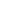How to calculate static moment?To know how to calculate the static torque, let's review the definition of the static torque first: when the object has a rotation trend on an axis but does not rotate, the torque generated is the static distance.

Calculation method of static moment:
< definition > if the distance between a particle with mass m and a rotating axis L is l, the static torque of the particle to the rotating axis L is: m (L) =m × l
From the above relationship, suppose that a two-dimensional plate has a static moment on a rotating shaft, then
The quality element is: DM= ρ (x,y)ds
The static distance element is: DM (L) =l ×ρ (l,L)ds
The static moment of the plane to a rotating shaft is: m (L) = ∫ ∫ [s]l ×ρ (l,L)ds
Where s is the area of the two-dimensional plate, ρ (L, l) is the function of density with respect to position, and l is the moment of departure from the particle Mi perpendicular to the axis of rotation L.
Similarly, the static moment of a three-dimensional body on a plane can be defined: m (L) = ∫ ∫ ∫ [v]l ×ρ (l,L,z)dv
The above formula is the static moment of the object on the L-Z plane.

Theoretically, we know how to calculate the static torque, but in practical application, the selection of static torque needs to be determined according to the load of the motor. The load can be divided into inertia load and friction load. A single inertia load and a single friction load do not exist. When starting directly (usually at low speed), both kinds of loads should be considered. Inertia load is mainly considered when starting at accelerated speed, and friction load is only considered when running at constant speed. Generally, the static moment should be between 2-3 times of the friction load. Once the static moment is selected, the base and length of the motor can be determined. If you are not sure how much static torque you need to use, or do not know how to calculate the static torque, you can contact our colleagues, and we will have professional engineers guide you.WhatsApp QR codeChat with robot Hermione
already 1 902 messages• Hermione 11:25.AM  Sep.13,2022
Hello, dear sir/madam, welcome to our website! I’m Hermione, how should I address you?